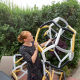# #MathArtChallenge Day 67: Circular Celtic Grid

The challenge: To create this circular grid and then design using it.

Materials needed: Compass OR Desmos geometry.
Math concepts you could explore with this challenge: circles, proportions/ratios, symmetry

Instructions are here: https://design.tutsplus.com/tutorials/geometric-design-a-celtic-grid–cms-24560

If you don’t have a compass, you could print out this pdf from my Desmos geometry construction:

Depending on how you use this activity, you may engage with different mathematical standards. I’ve listed possible connected math content above. Here are a few suggestions for how you might integrate the 8 mathematical practices. Feel free to add your own suggestions in the comments!

1.) Make sense of problems and persevere in solving them. Describe the proportion of each circle with respect to the others. Is that the same or different as the ratio between the circumferences?

7.) Look for and make use of structure. Without having lines intersect each other, what is the largest number of arcs (creating areas with space) and the fewest number of arcs possible?## Author: Ms. P

Math Teacher in Minneapolis, MN.